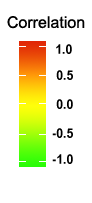STABILITYSOFT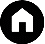STABILITYSOFT calculates the several parametric and non-parametric statistics as defined in STABILITYSOFT: a new online program to calculate parametric and non-parametric stability statistics for crop traits.Citation
Pour-Aboughadareh, A., M. Yousefian, H. Moradkhani, P. Poczai, and K. H. M. Siddique. 2019. STABILITYSOFT: A new online program to calculate parametric and non-parametric stability statistics for crop traits. Applications in Plant Sciences 7(1): e1211. doi:10.1002/aps3.1211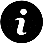Instructions

2. Click on the “Choose File“ button and select your data file.
3. Click “Get Results”.

Input Type:

 Pearson's correlation Type: Square Circle Mixed values and circle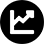Statistics

 Statistic Symbol Definition Mean variance component θi Plaisted and Peterson (1959) proposed the variance component of genotype environment interactions for interactions between each of the possible pairs of genotypes and considered the average of the estimate for all combinations with a common genotype to be a measure of stability. Accordingly, the genotypes which show lower value for the 𝜃𝑖 are considered more stable. GE variance component θ(i) This statistic is a modified measure of stability parameter. In this approach, ith genotype is deleted from the entire set of data and the GEI variance from this subset is the stability index for ith genotype. According to this statistic, the genotypes that show higher values for the θ(i) are considered more stable. Wricke’s ecovalence Wi2 Wricke (1962) proposed the concept of ecovalence as the contribution of each genotype to the GE interaction sum of squares. The ecovalence (Wi) of the ith genotype is its interaction with the environments, squared and summed across environments. Thus, genotypes with low values have smaller deviations from the mean across environments and are more stable. Regression coefficient *bi The slope regression (bi) is the response of the genotype to the environmental index that is derived from the average performance of all genotypes in each environment (Finlay and Wilkinson, 1963). If bi does not significantly differ from 1, then the genotype is adapted to all environments. A bi > 1 indicates genotypes with higher sensitivity to environmental change and greater specificity of adaptability to high-yielding environments, while a bi < 1 describes a measure of greater resistance to environmental change, thereby increasing the specificity of adaptability to low-yielding environments. Deviation from regression S2di In addition to slope regression, variance of deviations from the regression (S2di) has been suggested as one of the most-used parameter for the selection of stable genotypes. Genotypes with an S2di = 0 would be most stable, while an S2di > 0 would indicate lower stability across all environments. Hence, genotypes with lower values are the most desirable. Shukla’s stability variance σ2i Shukla  (1972)  suggested  the  stability  variance  of  genotype  i  as  its variance across environments after the main effects of  environmental  means  have  been  removed. According to this statistic, genotypes with minimum values are intended to be more stable. Coefficient of variance CVi The coefficient of variation is suggested by Francis and Kannenberg (1987) as a stability statistic through the combination of the coefficient of variation, mean yield, and environmental variance. Genotypes with low CVi, low environmental variance (EV), and high mean yield are considered to be the most desirable. Huhn’s and Nassar and Huhn’s non-parametric statistics S(1)S(2)S(3)S(6) Huhn (1990) and Nassar and Huhn (1987) suggested four non-parametric statistics: (1) S (1), the mean of the absolute rank differences of a genotype over all tested environments, (2) S (2), the variance among the ranks over all tested environments, (3) S (3), the sum of the absolute deviations for each genotype relative to the mean of ranks, and (4) S (6), the sum of squares of rank for each genotype relative to the mean of ranks. To compute these statistics, the mean yield data have to be transformed into ranks for each genotype and environment, and the genotypes are considered stable if their ranks are similar across environments. The lowest value for each of these statistics reveals high stability for a certain genotype. Thennarasu’s non-parametric statistics NP(1)NP(2)NP(3)NP(4) Four NP(1–4) statistics are a set of alternative non-parametric stability statistics defined by Thennarasu (1995). These parameters are based on the ranks of adjusted means of the genotypes in each environment. Low values of these statistics reflect high stability. Kang’s rank-sum Kang or KR Kang’s rank-sum (Kang, 1988) uses both yield and σ2i as selection criteria. This parameter gives a weight of one to both yield and stability statistics to identify high-yielding and stable genotypes. The genotype with the highest yield and lower σ2i are assigned a rank of one. Then, the ranks of yield and stability variance are added for each genotype and the genotypes with the lowest rank-sum are the most desirable. * To determine stability using this parameter, the significance test (H0: B≠1) must be conducted. For more detail, see the article by Finlay and Wilkinson (1963). ** In addition to S(i) statistics, two significance tests for Si(1) and Si(2), namely Z1 and Z2, will be calculated.

The formulae related to these calculations can be found in the original papers mentioned in the reference section.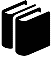Reference

Eberhart SAT and Russell WA (1966). Stability parameters for comparing varieties. Crop Science 6:36–40.

Finlay KW and Wilkinson GN (1963). Adaptation in a plant breeding programme. Australian Journal of Agricultural Research 14:742–754.

Francis TR and Kannenberg LW (1978). Yield stability studies in short-season maize: I. A descriptive method for grouping genotypes. Canadian Journal of Plant Science 58:1029–1034.

Huhn M (1990). Nonparametric measures of phenotypic stability. Part 1: Theory. Euphytica 47:189–1990.

Kang MS (1988). A rank-sum method for selecting high-yielding, stable corn genotypes. Cereal Research Communication 16:113–115.

Nassar R, and Huhn M (1987). Studies on estimation of phenotypic stability: tests of significance for nonparametric measures of phenotypic stability. Biometrics 43:45–53.

Plaisted RI, and Peterson LC (1959). A technique for evaluating the ability of selection to yield consistently in different locations or seasons. American Potato Journal 36:381–385.

Plaisted RL (1960). A shorter method for evaluating the ability of selections to yield consistently over locations. American Potato Journal 37:166–172.

Shukla GK (1972). Some statistical aspects of partitioning genotype-environmental components of variability. Heredity 29:237–245.

Thennarasu K (1995). On certain non-parametric procedures for studying genotype-environment interactions and yield stability. PhD thesis, PJ School, IARI, New Delhi, India.

Wricke G (1962). Übereine Methode zur Erfassung der ökologischen Streubreite in Feldversuchen. Zeitschrift für Pflanzenzüchtung 47:92–96.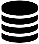The example data set files can be downloaded here.The codes written in R can be downloaded here.a.poraboghadareh@gmail.com or contact@mohsenyousefian.com